TitleCollege Algebra
Answer/Discussion to Practice Problems
Tutorial 2: Integer ExponentsAnswer/Discussion to 1a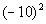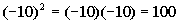*Write the base -10 in a product 2 times *MultiplyAnswer/Discussion to 1b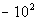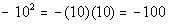*Negate 10 squared *Put a - in front of 10 written in a product 2 times *MultiplyAnswer/Discussion to 1c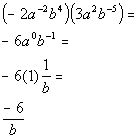*When mult. like bases you add your exponents: -2 + 2 = 0 and 4 + (-5) = -1

 Be careful here.  It is real tempting to take the reciprocal of -6 because of the negative sign.  Remember that you take the reciprocal of the base if the EXPONENT is negative not if the base is negative.Answer/Discussion to 1d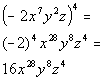Answer/Discussion to 1e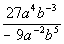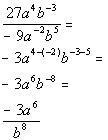Be careful here.  It is real tempting to take the reciprocal of -3 because of the negative sign.  Remember that you take the reciprocal of the base if the EXPONENT is negative not if the base is negative.Answer/Discussion to 1f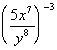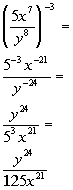Last revised on Dec. 4, 2009 by Kim Seward.
All contents copyright (C) 2002 - 2010, WTAMU and Kim Seward. All rights reserved.